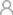ADAYO lighting as downlight supplier and this led recessed downlight has module design and high luminous efficiency up to 100lm/w

# What is “Power Factor” meaning?

Published byADAYOLIGHTING2023-01-23

Power factor is the ratio of the active power to the apparent power of an AC circuit. The more efficient and effective this value is for a given voltage and power of the user's electrical equipment, the better the power generation equipment can make full use of it. It is often expressed as cosΦ.

The power factor characterizes the ability of the lighting fixture to output active power. Power is a measure of the rate of energy transfer, and in a DC circuit it is the product of the voltage V and the current A. In the AC system it is more complicated, i.e. there is a part of AC current circulating in the load that does not transmit electrical energy, it is called reactance current or harmonic current, which makes the apparent power greater than the actual power.The size of the power factor (Power Factor) and the nature of the circuit load, such as incandescent bulbs, resistance furnace and other resistive load power factor of 1, the general inductive load circuit power factor is less than 1. The power factor is an important technical data of the power system. Power Factor is a factor that measures the efficiency of electrical equipment. A low power factor means that the circuit has a high reactive power for alternating magnetic field conversion, which reduces the utilization of the equipment and increases the loss of line power.

Take an example of a device. For example, the appliance has 100 units of power, which means that 100 units of power are delivered to the appliance. However, due to the inherent reactive power losses in most electrical systems, only 70 units of power can be used, in this example, the power factor is 0.7.

Each motor system consumes two large powers, the true active power (in watts) and the reactive reactive power (in watts). Power factor is the ratio between useful power and total power. The higher the power factor, the greater the ratio between useful power and total power, and the more efficient the system operation.

Most of the electrical loads in the grid, such as motors, transformers, fluorescent lamps and electric arc furnaces, are inductive loads. These inductive devices need to absorb not only active power but also reactive power from the power system during operation.Therefore, the installation of shunt capacitor reactive power compensation equipment in the grid will provide compensation for the reactive power consumed by the inductive loads, reducing the reactive power supplied by the power side of the grid to the inductive loads and transmitted by the lines. Since the flow of reactive power in the grid is reduced, the power loss of transformers and buses in transmission and distribution lines due to the transmission of reactive power can be reduced, which is the benefit of reactive power compensation.

Online message

Contact details86-752-5300601

Contact details

We hope to be your LED lighting R&D department